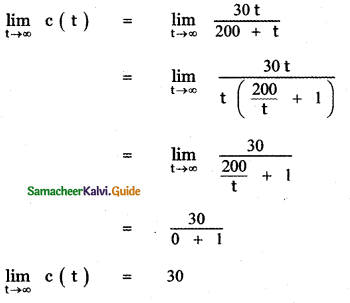Tamilnadu State Board New Syllabus Samacheer Kalvi 11th Maths Guide Pdf Chapter 9 Limits and Continuity Ex 9.3 Text Book Back Questions and Answers, Notes.

## Tamilnadu Samacheer Kalvi 11th Maths Solutions Chapter 9 Limits and Continuity Ex 9.3

Question 1.
(a) Find the left and right limits of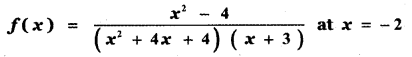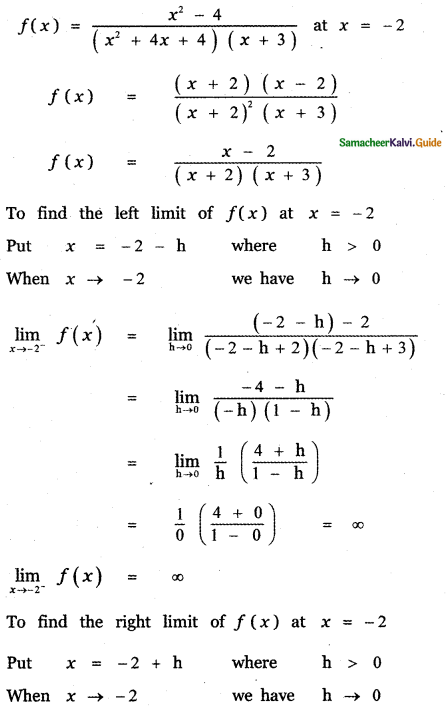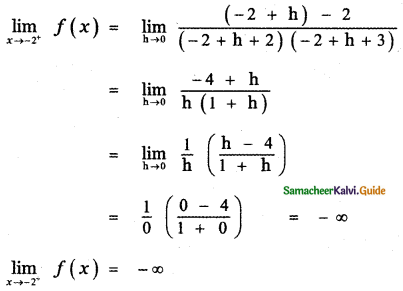(b) f(x) = tan x at x = $$\frac{\pi}{2}$$
To find the left limit of f(x) at x = $$\frac{\pi}{2}$$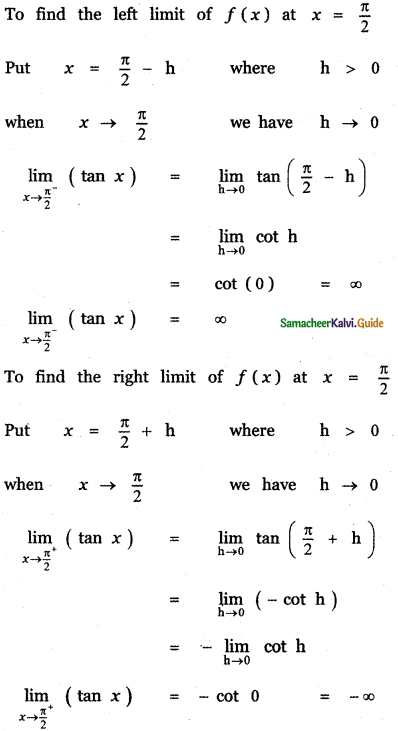Evaluate the following limits.

Question 2.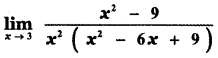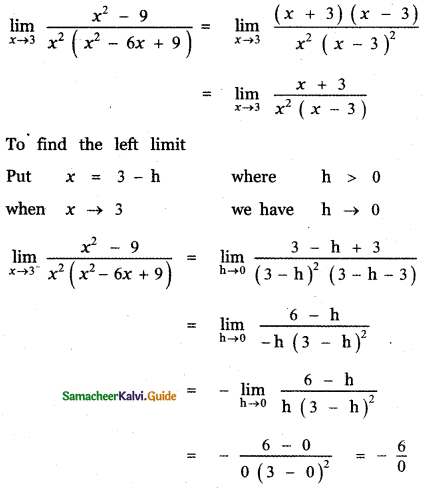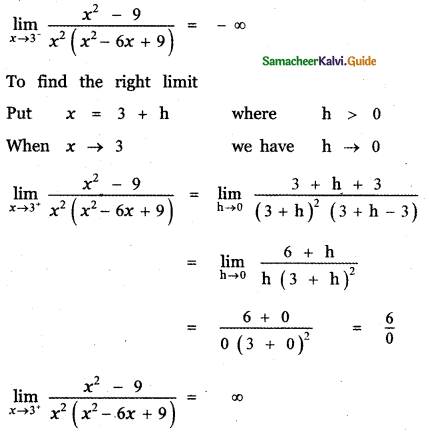Question 3.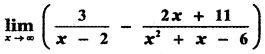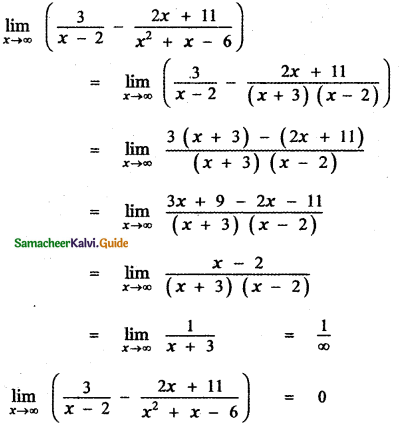Question 4.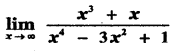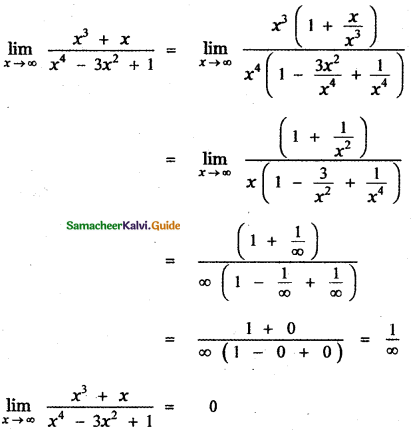Question 5.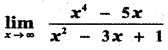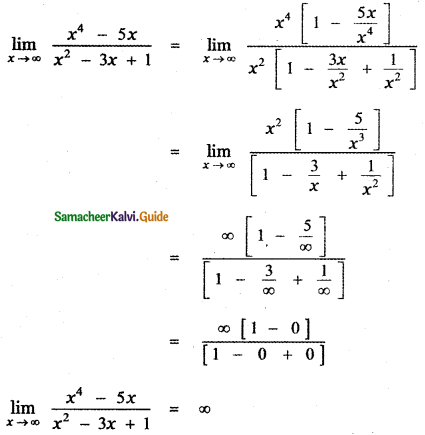Question 6.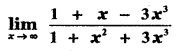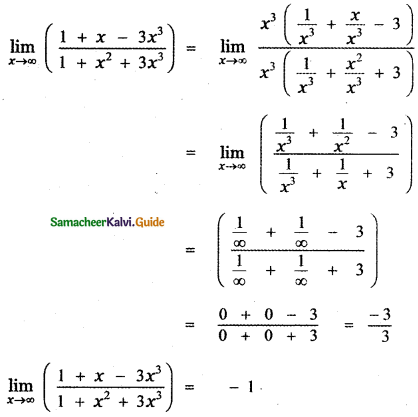Question 7.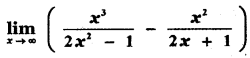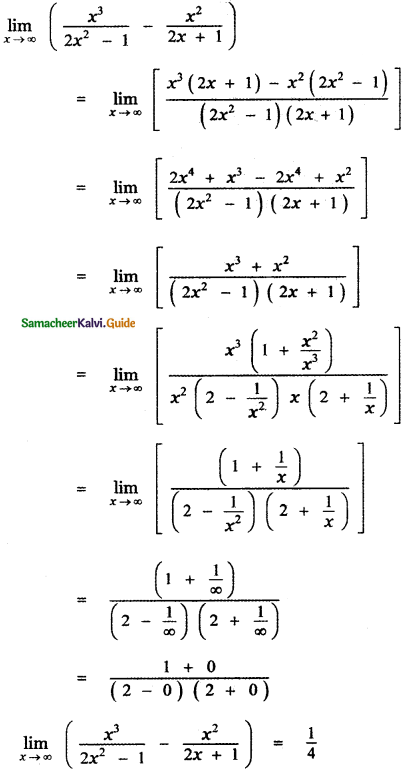Question 8.
Show that
(i)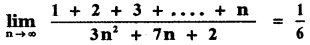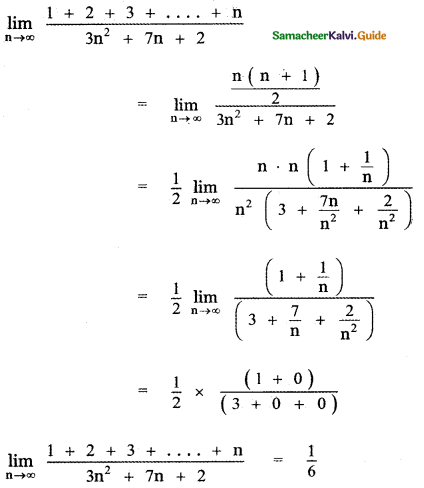(ii)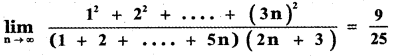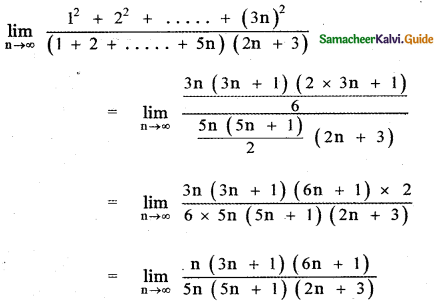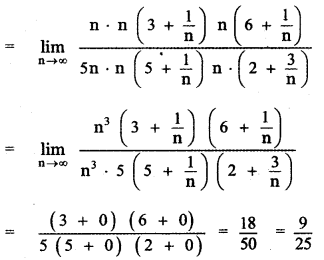(iii)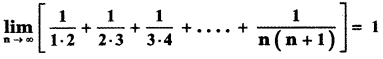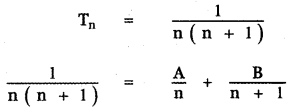1 = A(n + 1) + Bn
Put n = 0
1 = A (0 + 1) + B × 0
A = 1
Put n = -1
1 = A (-1 + 1) + B (-1)
1 = 0 – B ⇒ B = -1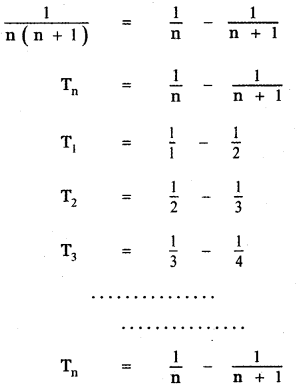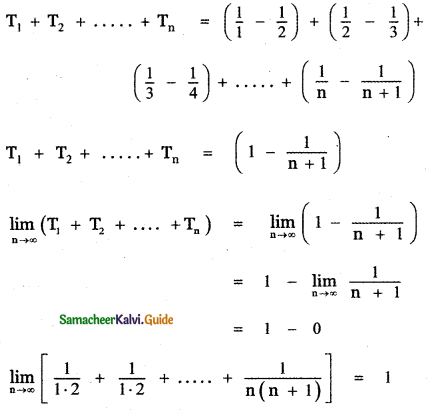Question 9.
An important problem in fishery science is to estimate the number of fish presently spawning in streams and use this information to predict the number of mature fish or “recruits” that will return to the rivers during the reproductive period. If S is the number of spawners and R the number of recruits, “Beverton – Holt spawner recruit function” is R(S) = $$\frac{\mathbf{s}}{(\alpha \mathbf{S}+\beta)}$$ where α and β are positive constants. Show that this function predicts approximately constant recruitment when the number of spawners is sufficiently large.
Given R(s) = $$\frac{\mathbf{s}}{(\alpha \mathbf{S}+\beta)}$$
where S is the number of spawners, R is the number of recruits. α, β are positive constants.
When the number of spawners is sufficiently large s → ∞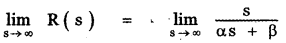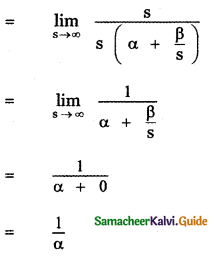When the number of spawners is sufficiently large, the number of recruits is $$\frac{1}{\alpha}$$

Question 10.
A tank contains 5000 litres of pure water. Brine (very salty water) that contains 30 grams of salt per litre of water is pumped into the tank at a rate of 25 litres per minute. The concentration of saltwater after t minutes (in grams per litre) is C(t) = $$\frac{30 t}{200+t}$$ What happens to the concentrations as t → ∞
C(t) = $$\frac{30 t}{200+t}$$